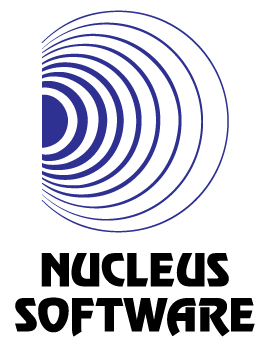Maximize Array Score
Tag(s):

Bitmask, Dynamic programming, Hard

Problem
Editorial
Analytics

Consider the following function which takes two integers P,Q and an array as input and returns the score of the array.

function Score(int P, int Q, int arr[]){
if(arr contains duplicate elements) return 0;
int ret = 0;
int n = arr.size();

for(int i=0;i<n;i++){
ret += pow(P,arr[i])%Q;
}
return ret;
}

You are given the integers P and Q and N integer arrays. You need to find the maximum possible value the above mentioned function can return, upon merging a maximum of 2 arrays among the given arrays.

Input

The first line contains three space separated integers N, P and Q

Each of the next N lines describe a single array. The first integer on each of those lines contains a single integer X denoting the size of the array. The next X integers represent the elements of the array.

Output:

Print the required answer on a single line.

Constraints

$1 \le N \le 100000$

$1 \le P \le 10000$

$1 \le Q \le 100000$

$\le 1 \le S_i \le 18$ , where $S_i$ represents the size of the $i^{th}$ array.

All the elements in the arrays are between $[1-18]$ inclusive

SAMPLE INPUT
3 5 7
3 1 2 3
2 3 4
3 4 5 6
SAMPLE OUTPUT
21
Explanation

The maximum possible return of the score function can be obtained by concatenating the first and the third arrays among the given ones, and then calling it.

Time Limit: 2.0 sec(s) for each input file.
Memory Limit: 256 MB
Source Limit: 1024 KB
Marking Scheme: Marks are awarded when all the testcases pass.
Allowed Languages: Bash, C, C++, C++14, Clojure, C#, D, Erlang, F#, Go, Groovy, Haskell, Java, Java 8, JavaScript(Rhino), JavaScript(Node.js), Julia, Kotlin, Lisp, Lisp (SBCL), Lua, Objective-C, OCaml, Octave, Pascal, Perl, PHP, Python, Python 3, R(RScript), Racket, Ruby, Rust, Scala, Swift, Swift-4.1, TypeScript, Visual Basic

CODE EDITOR

Initializing Code Editor...

This Problem was Asked inChallenge Name

Nucleus Software Coding Challenge

OTHER PROBLEMS OF THIS CHALLENGE
• Algorithms > Searching
• Math > Number Theory